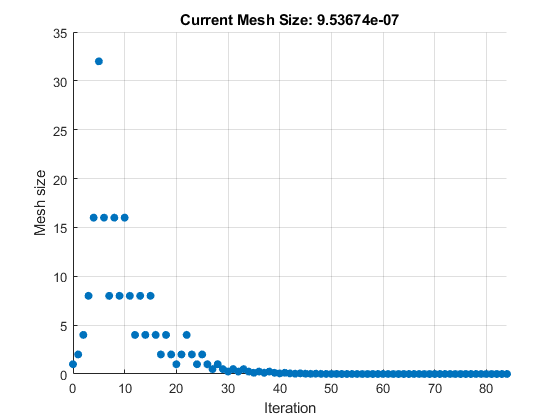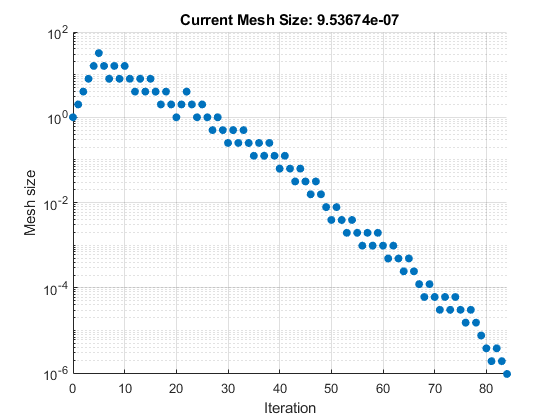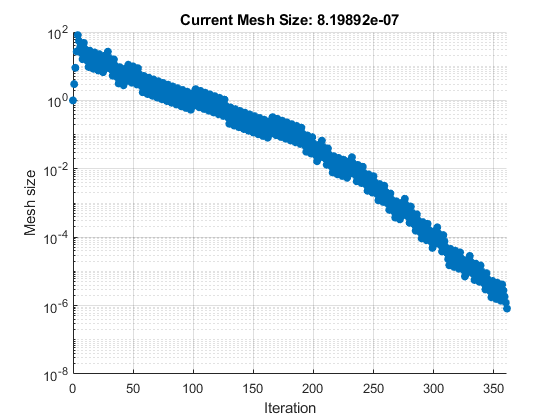## Set Mesh Options

### Mesh Expansion and Contraction

The Expansion factor and Contraction factor options, in Mesh options, control how much the mesh size is expanded or contracted at each iteration. With the default Expansion factor value of `2`, the pattern search multiplies the mesh size by `2` after each successful poll. With the default Contraction factor value of `0.5`, the pattern search multiplies the mesh size by `0.5` after each unsuccessful poll.

You can view the expansion and contraction of the mesh size during the pattern search by selecting Mesh size in the Plot functions pane. To also display the values of the mesh size and objective function at the command line, set Level of display to `Iterative` in the Display to command window options.

For example, set up the problem described in Linearly Constrained Problem as follows:

1. Enter the following at the command line:

```x0 = [2 1 0 9 1 0]; Aineq = [-8 7 3 -4 9 0]; bineq = 7; Aeq = [7 1 8 3 3 3; 5 0 -5 1 -5 8; -2 -6 7 1 1 9; 1 -1 2 -2 3 -3]; beq = [84 62 65 1];```
2. Set up your problem in the Optimization app to match the following figures.3. Run the optimization.The Optimization app displays the following plot.To run this problem at the command line, set options and run the problem as follows.

```x0 = [2 1 0 9 1 0]; Aineq = [-8 7 3 -4 9 0]; bineq = 7; Aeq = [7 1 8 3 3 3; 5 0 -5 1 -5 8; -2 -6 7 1 1 9; 1 -1 2 -2 3 -3]; beq = [84 62 65 1]; options = optimoptions('patternsearch',... 'PollMethod','GSSPositiveBasis2N',... 'PlotFcn',@psplotmeshsize,... 'Display','iter'); [x,fval,exitflag,output] = patternsearch(@lincontest7,x0,... Aineq,bineq,Aeq,beq,[],[],[],options);```

To see the changes in mesh size more clearly, change the y-axis to logarithmic scaling as follows:

1. Select Axes Properties from the Edit menu in the plot window.

2. In the Properties Editor, select the Y Axis tab.

3. Set Scale to Log.

Updating these settings in the MATLAB® Property Editor shows the plot in the following figure.The first 5 iterations result in successful polls, so the mesh sizes increase steadily during this time. You can see that the first unsuccessful poll occurs at iteration 6 by looking at the command-line display:

```Iter f-count f(x) MeshSize Method 0 1 2273.76 1 1 2 2251.69 2 Successful Poll 2 3 2209.86 4 Successful Poll 3 4 2135.43 8 Successful Poll 4 5 2023.48 16 Successful Poll 5 6 1947.23 32 Successful Poll 6 15 1947.23 16 Refine Mesh```

Note that at iteration 5, which is successful, the mesh size doubles for the next iteration. But at iteration 6, which is unsuccessful, the mesh size is multiplied `0.5`.

To see how Expansion factor and Contraction factor affect the pattern search, make the following changes:

• Set Expansion factor to `3.0`.

• Set Contraction factor to `2/3`.Then click . The Run solver and view results pane shows that the final point is approximately the same as with the default settings of Expansion factor and Contraction factor, but that the pattern search takes longer to reach that point.Alternatively, at the command line:

```options.MeshExpansionFactor = 3; options.MeshContractionFactor = 2/3; [x,fval,exitflag,output] = patternsearch(@lincontest7,x0,... Aineq,bineq,Aeq,beq,[],[],[],options);```

When you change the scaling of the y-axis to logarithmic, the mesh size plot appears as shown in the following figure.Note that the mesh size increases faster with Expansion factor set to `3.0`, as compared with the default value of `2.0`, and decreases more slowly with Contraction factor set to `0.75`, as compared with the default value of `0.5`.

### Mesh Accelerator

The mesh accelerator can make a pattern search converge faster to an optimal point by reducing the number of iterations required to reach the mesh tolerance. When the mesh size is below a certain value, the pattern search contracts the mesh size by a factor smaller than the Contraction factor factor. Mesh accelerator applies only to the GPS and GSS algorithms.

### Note

For best results, use the mesh accelerator for problems in which the objective function is not too steep near the optimal point, or you might lose some accuracy. For differentiable problems, this means that the absolute value of the derivative is not too large near the solution.

To use the mesh accelerator, set Accelerator to `On` in the Mesh options. Or, at the command line, set the `AccelerateMesh` option to `true`.

For example, set up the problem described in Linearly Constrained Problem as follows:

1. Enter the following at the command line:

```x0 = [2 1 0 9 1 0]; Aineq = [-8 7 3 -4 9 0]; bineq = 7; Aeq = [7 1 8 3 3 3; 5 0 -5 1 -5 8; -2 -6 7 1 1 9; 1 -1 2 -2 3 -3]; beq = [84 62 65 1];```
2. Set up your problem in the Optimization app to match the following figures.3. Run the optimization.Alternatively, at the command line enter

```x0 = [2 1 0 9 1 0]; Aineq = [-8 7 3 -4 9 0]; bineq = 7; Aeq = [7 1 8 3 3 3; 5 0 -5 1 -5 8; -2 -6 7 1 1 9; 1 -1 2 -2 3 -3]; beq = [84 62 65 1]; options = optimoptions('patternsearch',... 'PollMethod','GSSPositiveBasis2N',... 'Display','iter','AccelerateMesh',true); [x,fval,exitflag,output] = patternsearch(@lincontest7,x0,... Aineq,bineq,Aeq,beq,[],[],[],options);```

The number of iterations required to reach the mesh tolerance is 78, as compared with 84 when Accelerator is set to `Off`.

You can see the effect of the mesh accelerator by setting Level of display to `Iterative` in Display to command window. Run the example with Accelerator set to `On`, and then run it again with Accelerator set to `Off`. The mesh sizes are the same until iteration 70, but differ at iteration 71. The MATLAB Command Window displays the following lines for iterations 70 and 71 with Accelerator set to `Off`.

```Iter f-count f(x) MeshSize Method 70 618 1919.54 6.104e-05 Refine Mesh 71 630 1919.54 3.052e-05 Refine Mesh```

Note that the mesh size is multiplied by `0.5`, the default value of Contraction factor.

For comparison, the Command Window displays the following lines for the same iteration numbers with Accelerator set to `On`.

```Iter f-count f(x) MeshSize Method 70 618 1919.54 6.104e-05 Refine Mesh 71 630 1919.54 1.526e-05 Refine Mesh```

In this case the mesh size is multiplied by `0.25`.

Watch now